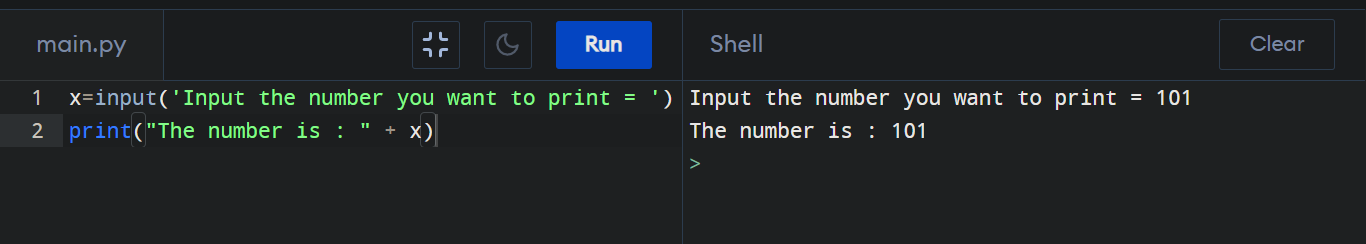Python program to print an user defined data of a integer variable

How to take an "int" type user input in Python? A user can take input using input function, and the number is printed on screen using print.

Python program to print user given input (Integer) :

x=input('Input the number you want to print = ')
print("The number is : " + x)

Output :